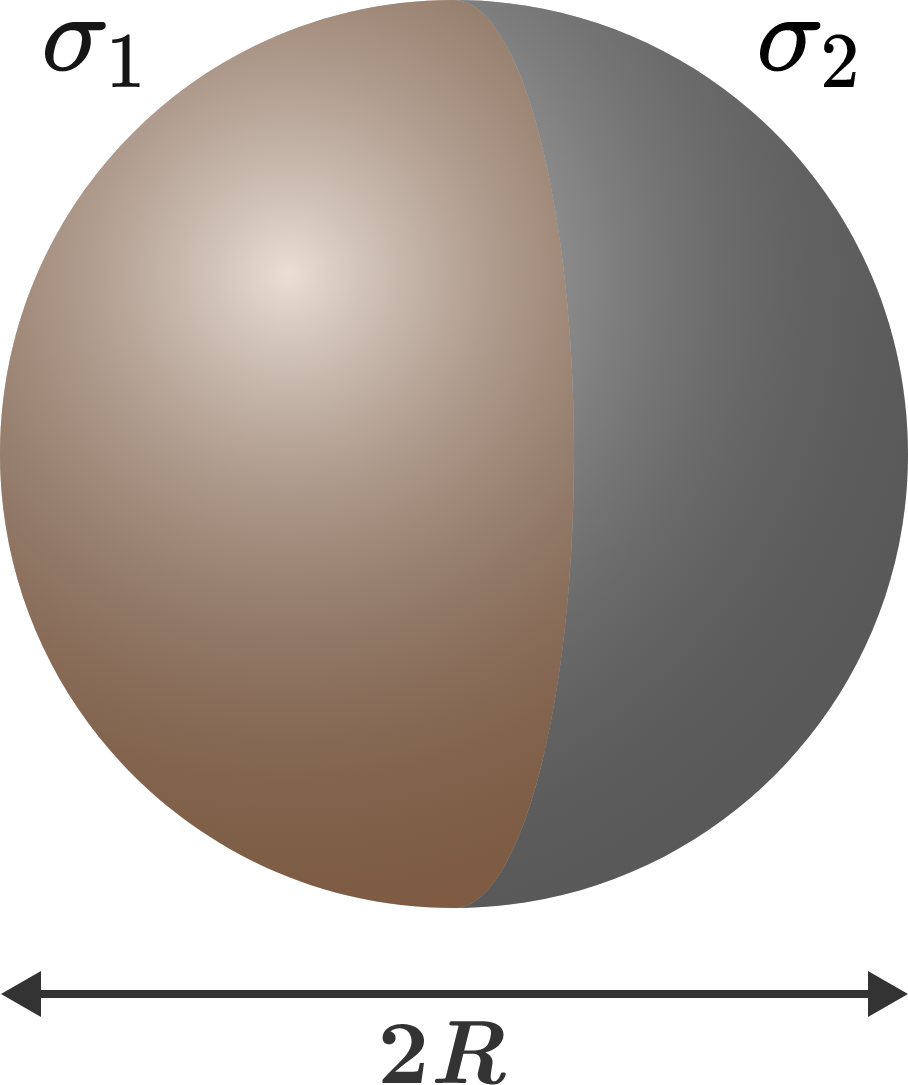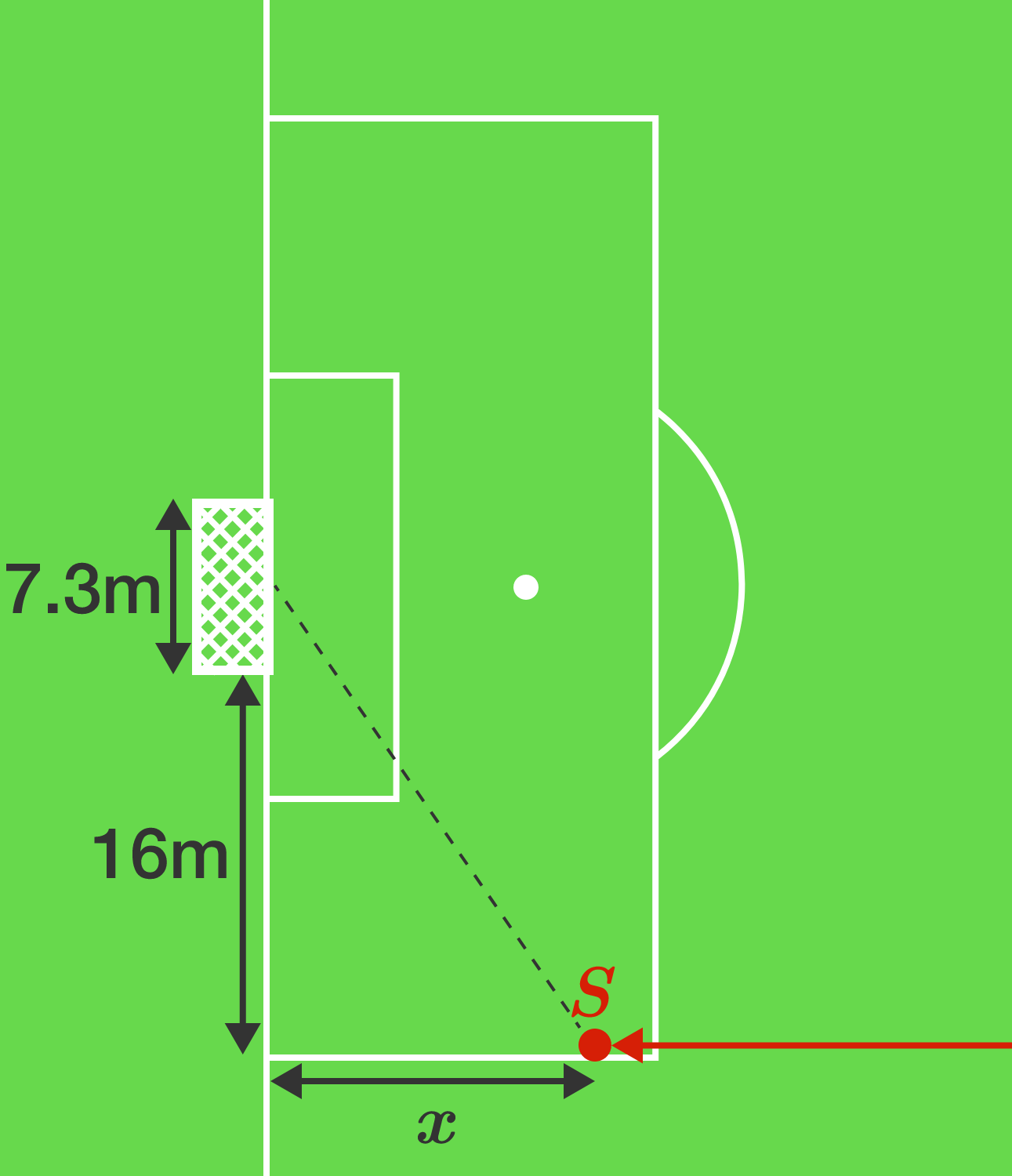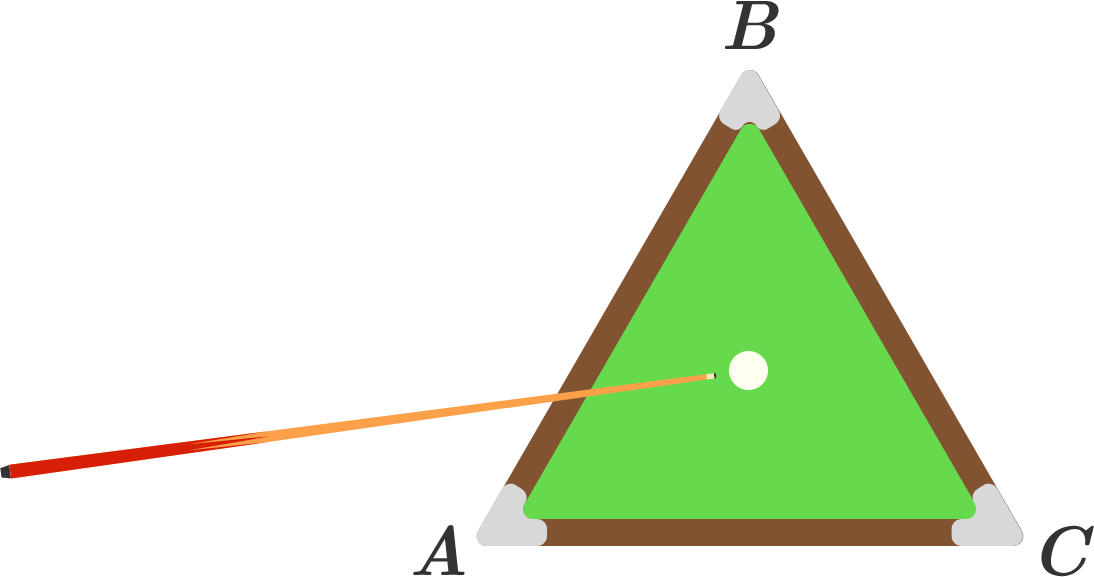# Problems of the Week

Contribute a problem

# 2017-12-04 Advanced

$2^{72} - 1, \quad 3^{72} - 1, \quad 4^{72} - 1,\quad \ldots , \quad 72^{72} - 1$

Find the greatest common divisor of the numbers above.Two half-spheres with fixed surface charge densities $\sigma_1$ and $\sigma_2$ and radius $R$ are brought into contact, as shown to the right.

Find the force of interaction between the two half-spheres.In a soccer match, the striker $($point $S)$ has beaten all defenders and is running parallel to the sideline towards the goal line. At what distance $x$ from the goal line should the striker shoot at the goal for the best chance of a goal? Give the result in meters and round to one decimal place.


Details and Assumptions:

• The striker will shoot the ball in a straight line on the ground.
• The striker isn't perfectly accurate, so his shot may be slightly off from where he intends.

You are playing pool on a billiard table which is an equilateral triangle $ABC.$ The ball is at the center of the triangle, and you hit it in any direction with uniform probability. What is the expected number of sides the ball hits before hitting side $\overline{AB},$ to 3 decimal places?Details and Assumptions:

• The ball bounces off the sides of the table according to the law of reflection.
• If the first side the ball hits is $\overline{AB},$ then the number of walls the ball hits before hitting $\overline{AB}$ is zero.
• The ball is infinitesimally small.

Let $S$ be a subset of $[0,1]$ consisting of a union of $10$ disjoint closed intervals $I_1, I_2, \ldots, I_{10}.$

Suppose $S$ has the property that for every $d \in [0,1],$ there are two points $x,y \in S$ such that $|x-y|=d.$

Letting $s = \sum\limits_{n=1}^{10} \text{length}(I_n),$ what is the minimum possible value of $s?$

Your answer should be a rational number $\frac{p}{q},$ where $p$ and $q$ are coprime positive integers.

Find $p+q.$

Bonus: Describe the sets $S$ for which the minimum is attained.

×

Problem Loading...

Note Loading...

Set Loading...